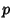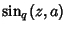## q-Cosine

The q-Analog of the Cosine function, as advocated by R. W. Gosper, is defined bywhereis a Theta Function andis defined viaThis is a period, Even Function of unit amplitude with double and triple angle formulas and addition formulas which are analogous to ordinary Sine and Cosine. For example,whereis the q-Sine, andis q-Pi. The-cosine also satisfiesGosper, R. W. Experiments and Discoveries in-Trigonometry.'' Unpublished manuscript.